## Suppose a term of a geometric sequence is a4 = 121.5 and the common ratio is 3. Write the formula for this sequence in the form an = a1 ⋅ rn

Question

Suppose a term of a geometric sequence is a4 = 121.5 and the common ratio is 3. Write the formula for this sequence in the form an = a1 ⋅ rn−1. Explain how you arrived at your answer.

in progress 0
5 months 2021-08-04T20:21:02+00:00 1 Answers 30 views 0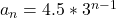Step-by-step explanation:

Given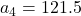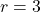Required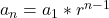Substitute 4 for n in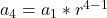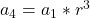Substitute 121.5 for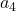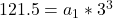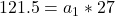Solve for a1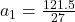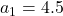So, we have: